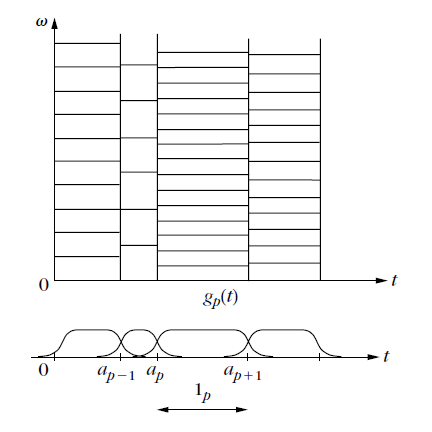# 0.5 Sparsity in redundant dictionaries

 Page 1 / 3
This collection comprises Chapter 1 of the book A Wavelet Tour of Signal Processing, The Sparse Way(third edition, 2009) by Stéphane Mallat. The book's website at Academic Press ishttp://www.elsevier.com/wps/find/bookdescription.cws_home/714561/description#description The book's complementary materials are available athttp://wavelet-tour.com

In natural languages, large dictionaries are needed to refine ideas with short sentences, and they evolve with usage.Eskimos have eight different words to describe snow quality , whereas a single word is typically sufficient in a Parisian dictionary.Similarly, large signal dictionaries of vectors are needed to constructsparse representations of complex signals. However, computing and optimizing a signal approximation by choosingthe best M dictionary vectors is much more difficult.A local cosine basis divides the time axis with smooth windows g p ( t ) and translates these windows into frequency.

## Frame analysis and synthesis

Suppose that a sparse family of vectors ${\left\{{\phi }_{p}\right\}}_{p\in \Lambda }$ has been selected to approximate a signal $\phantom{\rule{0.166667em}{0ex}}f$ . An approximation can be recoveredas an orthogonal projection in the space V λ generated by these vectors. We then face one of the following twoproblems.

1. In a dual-synthesis problem, the orthogonal projection $\phantom{\rule{0.166667em}{0ex}}{f}_{\lambda }$ of $\phantom{\rule{0.166667em}{0ex}}f$ in V λ must be computed from dictionary coefficients, ${\left\{⟨\phantom{\rule{0.166667em}{0ex}}f,{\phi }_{p}⟩\right\}}_{p\in \lambda }$ , provided by an analysis operator.  This is the case when a signal transform ${\left\{⟨\phantom{\rule{0.166667em}{0ex}}f,{\phi }_{p}⟩\right\}}_{p\in \Gamma }$ is calculated in some large dictionary and a subset of inner products are selected. Such inner products may correspond tocoefficients above a threshold or local maxima values.
2. In a dual-analysis problem, the decomposition coefficients of $\phantom{\rule{0.166667em}{0ex}}{f}_{\lambda }$ must be computed on a family of selected vectors ${\left\{{\phi }_{p}\right\}}_{p\in \Lambda }$ . This problem appears when sparse representation algorithmsselect vectors as opposed to inner products. This is the case forpursuit algorithms, which compute approximation supports in highly redundant dictionaries.

The frame theory gives energy equivalence conditions to solve both problems with stable operators.A family ${\left\{{\phi }_{p}\right\}}_{p\in \Lambda }$ is a frame of the space V it generatesif there exists $B\ge A>0$ such that

$\forall h\in {\mathbf{V},\phantom{\rule{5.0pt}{0ex}}A\parallel h\parallel }^{2}\phantom{\rule{0.166667em}{0ex}}\le \phantom{\rule{0.166667em}{0ex}}\sum _{m\in \lambda }|⟨h,{\phi }_{p}{⟩|}^{2}\phantom{\rule{0.166667em}{0ex}}\le \phantom{\rule{0.166667em}{0ex}}B{\parallel h\parallel }^{2}.$

The representation is stable since any perturbation of frame coefficientsimplies a modification of similar magnitude on h . Chapter 5 proves that the existence of a dual frame ${\left\{{\stackrel{˜}{\phi }}_{p}\right\}}_{p\in \Lambda }$ that solves both the dual-synthesis and dual-analysisproblems:

$\begin{array}{c}\hfill {f}_{\lambda }=\sum _{p\in \lambda }⟨\phantom{\rule{0.166667em}{0ex}}f,{\phi }_{p}⟩\phantom{\rule{0.166667em}{0ex}}{\stackrel{˜}{\phi }}_{p}=\sum _{p\in \lambda }⟨\phantom{\rule{0.166667em}{0ex}}f,{\stackrel{˜}{\phi }}_{p}⟩\phantom{\rule{0.166667em}{0ex}}{\phi }_{p}.\end{array}$

Algorithms are provided to calculate these decompositions.The dual frame is also stable:

$\forall f\phantom{\rule{0.166667em}{0ex}}\in \phantom{\rule{0.166667em}{0ex}}\mathbf{V},\phantom{\rule{5.0pt}{0ex}}{B}^{-1}{\parallel \phantom{\rule{0.166667em}{0ex}}f\parallel }^{2}\le \sum _{m\in \gamma }|⟨\phantom{\rule{0.166667em}{0ex}}f,{\stackrel{˜}{\phi }}_{p}{⟩|}^{2}\phantom{\rule{0.166667em}{0ex}}\le \phantom{\rule{0.166667em}{0ex}}{B}^{-1}{\parallel \phantom{\rule{0.166667em}{0ex}}f\parallel }^{2}.$

The frame bounds A and B are redundancy factors. If the vectors ${\left\{{\phi }_{p}\right\}}_{p\in \Gamma }$ are normalized and linearly independent, then $A\le 1\le B$ . Such a dictionary is called a Riesz basis of V and the dual frame is biorthogonal:

$\forall \left(\phantom{\rule{0.166667em}{0ex}}p,p\text{'}\right)\in {\lambda }^{2},\phantom{\rule{3.33333pt}{0ex}}⟨{\phi }_{p},{\stackrel{˜}{\phi }}_{p\text{'}}⟩=\delta \left[\phantom{\rule{0.166667em}{0ex}}p-p\text{'}\right].$

When the basis is orthonormal, then both bases are equal. Analysis and synthesis problems are then identical.

The frame theory is also used to construct redundant dictionaries that define complete, stable, and redundant signal representations,where V is then the whole signal space. The frame bounds measure the redundancy of such dictionaries.Chapter 5 studies the construction of windowed Fourier and wavelet frame dictionaries by samplingtheir time, frequency, and scaling parameters, while controlling frame bounds.In two dimensions, directional wavelet frames include wavelets sensitive to directional image structures such as textures or edges.

A closed circulatory system is a closed-loop system, in which blood is not free in a cavity. Blood is separate from the bodily interstitial fluid and contained within blood vessels. In this type of system, blood circulates unidirectionally from the heart around the systemic circulatory route, and th
food
Ramatulai
what is meiosis
meiosis is a reduction Division in which four hapliod off springs are produced
Nandanee
what is mitosis?
(cytology) the division of cell nucleus in which the genome is copied and separated into two identical halves.it is normally follow by cell division
it Is the division of the mother cell into two daughter cells,genetically identical to each other and to their parent cell
Joneth
it is a study of living organisms
What are other types of cell
plant and animal cell
Jessy
prokaryotic and eukaryotic cell
Joneth
meaning inside and outside cells
Joneth
external feature of earthworm
what is the reason behind that before F2 generation is no segregation?
what is mitosis and meiosis
hypothesis theory law
what is hypothesis theory law
Tamba
Briefly describe the process of mitosis and meiosis.
what is chromosome
Chromosome is the thread-like structure containing DNA and found in the nucleus of a cell. DNA contains gene which has genetic information.
Lilian
if a cell is killed by strain why then is it use
what is an atom
an atom is the must smallest element in the world.
Young
please help me friends. I wont a good example of lab report based on carbohydrate,lipid,reducing and non reducing sugar.
Ng
that can't be split by any chemical means
uchenna
No an atom is the smallest particles of an element which can take part in a chemical reaction
Solayemi
An atom is the smallest indivisible particle of an element which can take part in a chemical reaction.
Samuel
sure atoms can't be splited
Solayemi
atom can't be split
john
what is molecule
john
Molecules consists of one or more tiny particles called atom
Rofiah
atoms joined together by covalent bonds
an atom is a particle that cannot be divided further by a chemical reaction
how do earthworms excrete
Ndlovu
what is genotype
Got questions? Join the online conversation and get instant answers!ByByBy